# Higher-Order Partial Derivatives Definition & Examples

Lesson Transcript
Instructor: Gerald Lemay

Gerald has taught engineering, math and science and has a doctorate in electrical engineering.

In this lesson, we define the partial derivative and then extend this concept to find higher-order partial derivatives. Examples are used to expand your knowledge and skill.

## Review of Partial Derivatives

Fred has mastered differentiating when a function has only one variable. Somehow, he missed the lessons on partial differentiation. He's starting to panic because the current homework deals with higher-order partial derivatives.

In this lesson, we review partial differentiation by using examples. Then, we extend the method to higher-order partial derivatives. Let's zoom in on Fred to see what he's currently trying to figure out.

Our fearless math student, Fred, is being asked to find the derivative of x4 - x3. He correctly answers: 4x3- 3x2. Let's help Fred with his review of partial derivatives. You ask him to find the derivative of x4a - x3b where a and b are constants. Again, a correct answer: 4x3a- 3x2b.

Now the fun begins. Let's read the following math statement: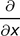It says: the partial (derivative) with respect to x. The word ''derivative'' is in parentheses because we often just say: the partial with respect to x.

Let's write the derivative of x4a - x3b as a partial derivative: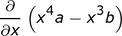The answer is still 4x3a- 3x2b.

The a and the b are constants. When taking the partial derivative with respect to x, everything other than x is a constant. So we could write: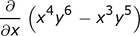and y6 and y5 are treated as constants. So, the answer is still the same except the a and b constants are more specific. The answer is now x4y6 - x3y5. Thus, if f(x, y) is the function: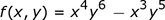and y6 and y5 are treated as constants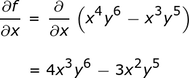Of course, we can also have a partial with respect to y where everything else is constant. To do these kinds of derivatives, you develop a selective focus with your eyes. You ''see'' the y as the variable and the x4 and the x3 as constants.

Thus,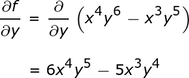And this is pretty much the idea with partial derivatives. You just have to be careful with the ''with respect to'' part. Fred is ready to move on to higher-order derivatives.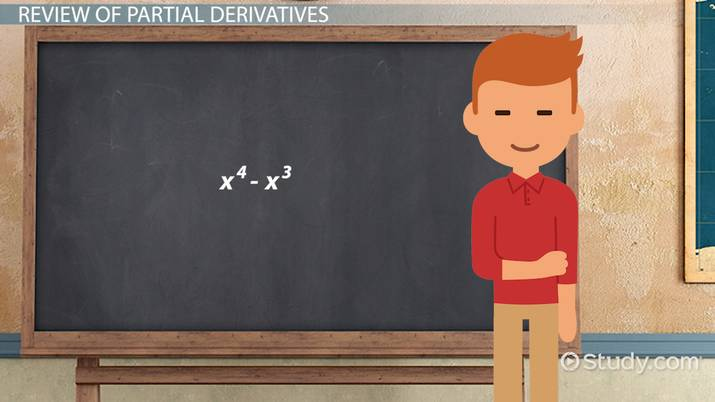An error occurred trying to load this video.

Try refreshing the page, or contact customer support.

Coming up next: Tangent Plane to the Surface

### You're on a roll. Keep up the good work!

Replay
Your next lesson will play in 10 seconds
• 0:04 Review of Partial Derivatives
• 2:36 Second-Order Partials
• 4:03 Mixed Partial Derivatives
• 4:45 Example 1
• 5:24 Example 2
• 5:57 Lesson Summary
Save Save

Want to watch this again later?

Timeline
Autoplay
Autoplay
Speed Speed

## Second-Order Partials

Let's say we want to take the partial derivative of the partial derivative. We write this as: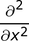and read this as the second partial with respect to x. This is called a second-order partial derivative. Using the example for f(x, y), here are the details: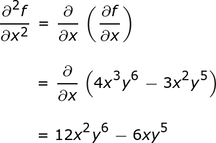We have:

• Line 1: The partial squared with respect to x squared of f with respect to x squared of f is the partial with respect to x of the partial of f with respect to x. We have already computed the quantity in parentheses.
• Line 2: Replace the quantity in parentheses with 4x3y6 - 3x2y5.
• Line 3: Take the derivative with respect to x gives 12x2y6 - 6xy5.

For practice, we ask Fred to work on the second partial with respect to y of f. The details: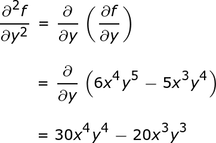This idea of the partial derivative of the partial derivative of the … can be extended to even higher-order derivatives.

Fred is overjoyed at his success with higher-order partial derivatives. But wait, there's one more item to check out.

To unlock this lesson you must be a Study.com Member.

### Register to view this lesson

Are you a student or a teacher?